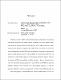## Non-local Transport Signatures and Quality Factors in the Realistic Majorana Nanowire2022
##### Authors
Lai, Yi-Hua
Majorana zero modes (MZMs) can be fault-tolerant topological qubits due to their topological protection property and non-Abelian statistics. Over the last two decades, a deluge of theoretical predictions and experimental observations has been actively ongoing in the hope of implementing topological quantum computation upon MZMs. Among several solid-state systems, the most promising platform to realize MZMs is the one-dimensional semiconductor-superconductor nanowire (called Majorana nanowire'' in short), which is the focused system in this thesis. It is fundamental to identify MZMs as qubits to construct topological quantum computers. Therefore, the signatures of MZMs become highly crucial for verification. However, the earlier theoretical works demonstrate that topologically trivial Andreev bound states (ABSs) can mimic the hallmarks of MZMs in various aspects but do not carry topological properties. As a result, distinguishing MZMs from ABSs becomes significantly pivotal in the study of Majorana nanowire. One of the signatures the author studied is the robustness of the quantized zero-bias conductance peak (ZBCP) in the realistic Majorana nanowire. The importance of this signature becomes further enhanced, particularly after the 2018 Nature paper, which displayed the quantized Majorana conductance, got retracted. In Chapter 2, the proposed quality factors quantify the robustness of quantized ZBCPs. By comparing the numerical results between different scenarios, this study shows that the quality factor $F$ can help distinguish topological MZMs from trivial subgap bound states in the low-temperature limit. Another necessary signature of MZMs is the non-local correlation. In Chapter 3, the conductance correlation is demonstrated by modeling the comparing quantum-point-contact (QPC) conductance from each end. Both the pristine nanowire and the quantum-dot-hybrid-nanowire system are modeled and compared, which shows the significance of non-local end-to-end correlation for the existence of MZMs. The other approach to simultaneously examining the localization of states at both ends of the nanowire is through the Coulomb blockade (CB) measurement. The lack of sensitivity to the localized state at only one end makes the CB spectroscopy able to capture the non-local correlation feature of MZMs. However, CB transport in the Majorana nanowire is much more complicated to analyze than QPC transport because (a) Coulomb interaction is treated as equal to MZM physics without perturbation, and (b) there are many energy levels in the nanowire, which gives rise to an exponential complexity to solve the rate equations. In Chapter 4, a generalized version of Meir-Wingreen formula for the tunneling conductance of a two-terminal system is derived. This formula reduces the exponential complexity of the rate equations to as low as the linear complexity of QPC tunneling, thus allowing multiple energy levels to be included in the calculation. With dominant realistic effects in the model, the experimental features, such as the bright-dark-bright CB conductance pattern and decreasing oscillation conductance peak spacings (OCPSs) with the Zeeman field, will be simulated and explained theoretically. In short, the theoretical methods proposed in this thesis, including the quality factors, non-local correlation ZBCPs, and CB spectroscopy, are intended to distinguish MZMs from other topologically trivial bound states. Further investigations on the robustness of quantized conductance and non-local correlation analysis can clarify the ambiguous signals in the experiments and push the realization of topological quantum computation to the frontier.